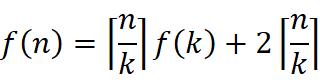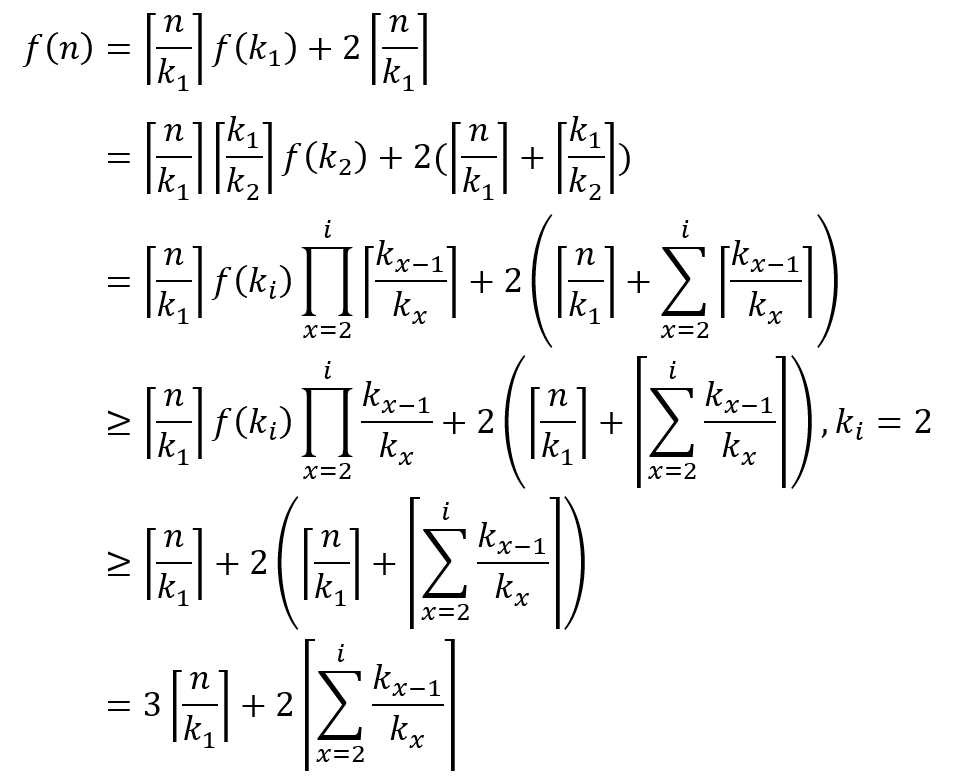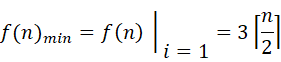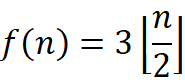# 算法基础-顺序统计量

## 顺序统计量

⌊n⌋ 表示对 n 向下取整，⌈n⌉表示对 n 向上取整

## 最大值和最小值

int min = a;
for(int i=1;i<n;i++){
if(a[i] > min)
min = a[i];
}

### 优化算法由于 kx 一定是正数，因此 i 为 1 时，右边的连加消失，f(n)取到最小值，即k1=2通过理论我们得知了只要把数组中的数两两比较，其中的较小者和最小值比较，而较大者和最大值比较，就可以实现用最少的比较次数同时求出最大最小值

void FindMinAndMax(int* a, int len){
int min, max, i;
if(len % 2 == 0){
//当长度为偶数时，将前两项之间的较大者设为最大值，较小者设为最小值
if(a < a){
min = a;
max = a;
}else{
min = a;
max = a;
}
//从a和a开始比较，i为3
i = 3;
}else{
//当长度为基数时，将最大值和最小值都设为a
min = max = a;
//从a和a开始比较，i为2
i = 2;
}
while (i < len){
if(a[i-1] < a[i]){
if(a[i-1]<min) min = a[i-1];
if(a[i]>max) max = a[i];
}else{
if(a[i]<min) min = a[i];
if(a[i-1]>max) max = a[i-1];
}
i += 2;
}
cout << "min:" << min << endl;
cout << "max:" << max << endl;
}

## 第i顺序统计量

int find(int *a, int left, int right, int k) {
if (left >= right) {
return a[left];
}
//key为选定的基准值
int i = left, j = right, key = a[left];
while (i < j) {
//遍历数组找到第一个比key大的数
while (a[i] < key) {
++i;
}
//遍历数组找到第一个比key小的数
while (a[j] > key) {
--j;
}
//交换，此时比key小的在左边，比key大的在右边
if (i < j) {
int temp = a[i];
a[i] = a[j];
a[j] = temp;
}
}
//此时基准值在整个数组的第i个位置
//s是基准值在以left开始的数组里的位置，i是基准值的位置
int s = i - left + 1;
if (s == k) {//如果s==k,说明基准值就是要查找的数,则返回基准值
return a[i];
} else if (s > k) {//如果s>k,说明基准值在要查找的数的右边,则遍历左边数组
return find(a, left, j, k);
} else {//如果s<k,说明基准值在要查找的数的左边,则遍历右边数组
return find(a, j + 1, right, k - s);
}
}

04-03
09-22681
04-16854
12-02952
03-15541
06-221万+
11-112056
06-22534
05-17227
07-153300
12-272963
07-241万+
03-22250
06-065937

### “相关推荐”对你有帮助么？

•非常没帮助
•没帮助
•一般
•有帮助
•非常有帮助被折叠的  条评论 为什么被折叠?到【灌水乐园】发言Dear_Xuan

¥1 ¥2 ¥4 ¥6 ¥10 ¥20获取中扫码支付点击重新获取扫码支付1.余额是钱包充值的虚拟货币，按照1:1的比例进行支付金额的抵扣。
2.余额无法直接购买下载，可以购买VIP、付费专栏及课程。余额充值